# HSSlive: Plus One & Plus Two Notes & Solutions for Kerala State Board

## BSEB Class 10 Maths Chapter 8 Introduction to Trigonometry Ex 8.4 Textbook Solutions PDF: Download Bihar Board STD 10th Maths Chapter 8 Introduction to Trigonometry Ex 8.4 Book AnswersBSEB Class 10 Maths Chapter 8 Introduction to Trigonometry Ex 8.4 Textbook Solutions PDF: Download Bihar Board STD 10th Maths Chapter 8 Introduction to Trigonometry Ex 8.4 Book Answers

BSEB Class 10th Maths Chapter 8 Introduction to Trigonometry Ex 8.4 Textbooks Solutions and answers for students are now available in pdf format. Bihar Board Class 10th Maths Chapter 8 Introduction to Trigonometry Ex 8.4 Book answers and solutions are one of the most important study materials for any student. The Bihar Board Class 10th Maths Chapter 8 Introduction to Trigonometry Ex 8.4 books are published by the Bihar Board Publishers. These Bihar Board Class 10th Maths Chapter 8 Introduction to Trigonometry Ex 8.4 textbooks are prepared by a group of expert faculty members. Students can download these BSEB STD 10th Maths Chapter 8 Introduction to Trigonometry Ex 8.4 book solutions pdf online from this page.

## Bihar Board Class 10th Maths Chapter 8 Introduction to Trigonometry Ex 8.4 Books Solutions

 Board BSEB Materials Textbook Solutions/Guide Format DOC/PDF Class 10th Subject Maths Chapter 8 Introduction to Trigonometry Ex 8.4 Chapters All Provider Hsslive

## How to download Bihar Board Class 10th Maths Chapter 8 Introduction to Trigonometry Ex 8.4 Textbook Solutions Answers PDF Online?

2. Click on the Bihar Board Class 10th Maths Chapter 8 Introduction to Trigonometry Ex 8.4 Answers.
3. Look for your Bihar Board STD 10th Maths Chapter 8 Introduction to Trigonometry Ex 8.4 Textbooks PDF.
4. Now download or read the Bihar Board Class 10th Maths Chapter 8 Introduction to Trigonometry Ex 8.4 Textbook Solutions for PDF Free.

## BSEB Class 10th Maths Chapter 8 Introduction to Trigonometry Ex 8.4 Textbooks Solutions with Answer PDF Download

Find below the list of all BSEB Class 10th Maths Chapter 8 Introduction to Trigonometry Ex 8.4 Textbook Solutions for PDF’s for you to download and prepare for the upcoming exams:

## BSEB Bihar Board Class 10th Maths Solutions Chapter 8 Introduction to Trigonometry Ex 8.4

Question 1.
Express the trigonometric ratios sin A, sec A and tan A in terms of cot A.
Solution:
Consider a ∆ ABC, in which ∠B = 90°.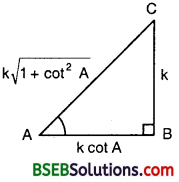Base = AB
Perp. = BC
and Hyp. = AC
∴ cot A = Base Perp = 𝐴𝐵𝐵𝐶
or 𝐴𝐵𝐵𝐶 = cot A = 𝑐𝑜𝑡𝐴1
Let AB = k cot A and BC = k.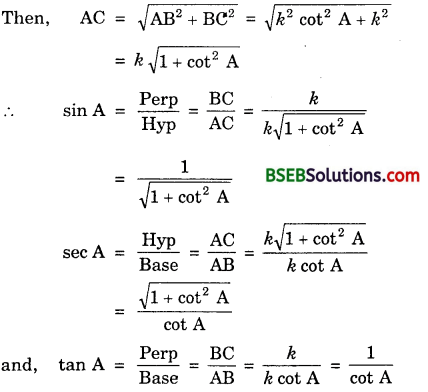Question 2.
Write the other trigonometric ratios of A in terms of sec A.
Solution:
Consider a ∆ ABC, in which ∠B = 90°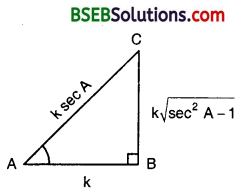For ∠A, we have :
Base = AB,
Perp = BC
Hyp = AC.
∴ sec A = Hyp Base = 𝐴𝐶𝐴𝐵
or 𝐴𝐶𝐴𝐵 = sec A = 𝑠𝑒𝑐𝐴1
Let AB = k and AC = k sec A.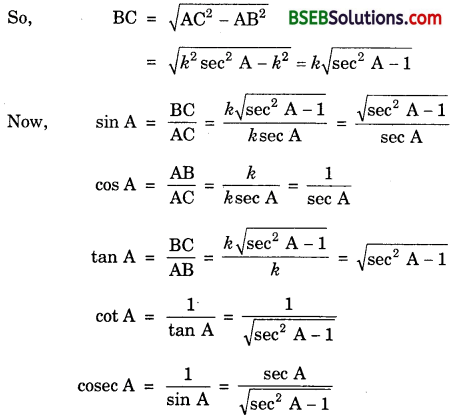Question 3.
Evaluate :
(i) sin263∘+sin227∘cos217∘+cos273∘
(ii) sin 25° cos 65° + cos 25° sin 65
Solution:
(i) Here, sin 63° = sin (90° – 27°) = cos 27°
and cos 17° = cos (90° – 73°) = sin 73°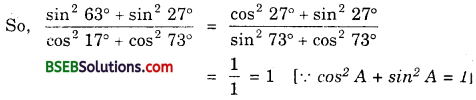(ii) sin 25° cos 65° + cos 25° sin 65°
= sin (90° – 65°) cos 65° + cos (90° – 65°) sin 65°
= cos 65° cos 65° + sin 65° sin 65°
= cos² 65° + sin² 65° = 1

Question 4.
Choose the correct option. Justify your choice :
(i) 9 sec² A – 9 tan² A =
(A) 1
(B) 9
(C) 8
(D) 0
(ii) (1 + tan θ + sec θ) (1 + cot θ – cosec θ) =
(A) 0
(B) 1
(C) 2
(D) none of these
(iii) (sec A + tan A)(1 – sin A) =
(A) sec A
(B) sin A
(C) cosec A
(D) cos A
(iv) 1+tan2𝐴1+cot2𝐴
(A) sec² A
(B) – 1
(C) cot² A
(D) none of these
Solution:
(i) (B), because
9 sec² A – 9 tan² A = 9 (sec² A – tan² A) = 9 x 1 = 9

(ii) (C), because
(1 + tan θ + sec θ )(1 + cot θ – cosec θ)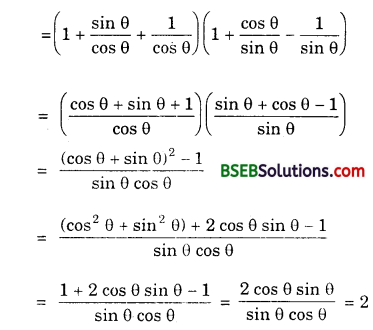(iii) (D), because
(sec A + tan A)(1 – sin A) =(iv) (D), becauseQuestion 5.
Prove the following identities, where the angles involved are acute angles for which the expressions are defined :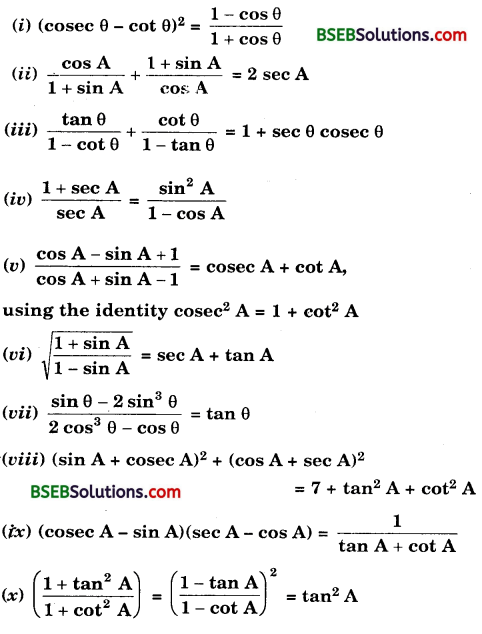Solution:
(i) We have :
L.H.S. = (cosec θ – cot θ)²

(ii) We have :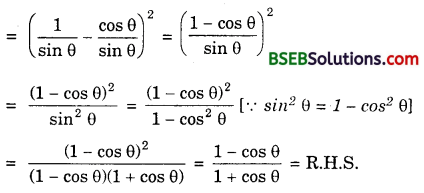(ii) We have :(iii) We have :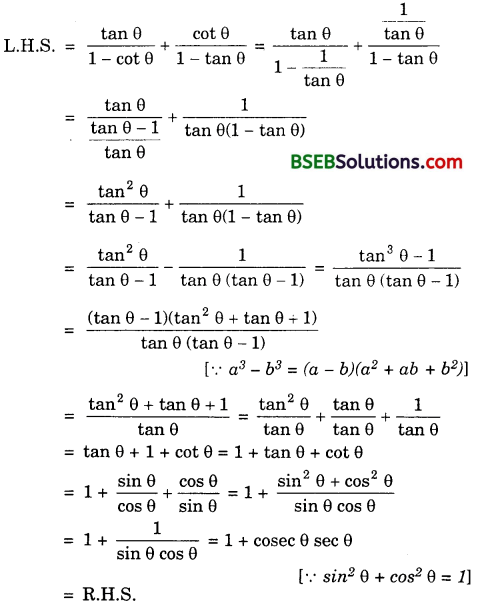(iv) We have :(v) We have :(vi) We have :(vii) We have :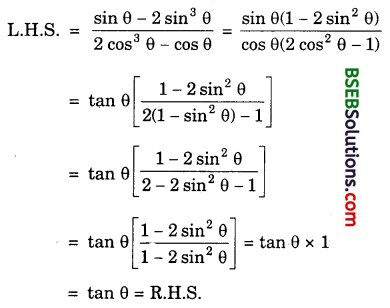(viii) We have :
L.H.S. = (sin A + cosec A)² + (cos A + sec A)²
= (sin² A + cosec² A + 2 sin A cosec A) + (cos² A + sec2 A + 2 cos A sec A)
= (sin² A + cosec² A + 2 sin A.1sin𝐴) + (cos² A + sec2 A + 2 cos A. 1cos𝐴)
= (sin²A + cosec² A + 2) + (cos² A + sec² A + 2)
= sin²A + cos² A + cosec² A + sec² A + 4
= 1 + (1 + cot² θ) + (1 + tan² A) + 7 + tan² A + cot² A [ ∵ cosec² A = 1 + cot² A and sec² A = 1 + tan² A]
= R.H.S.

(ix) We have :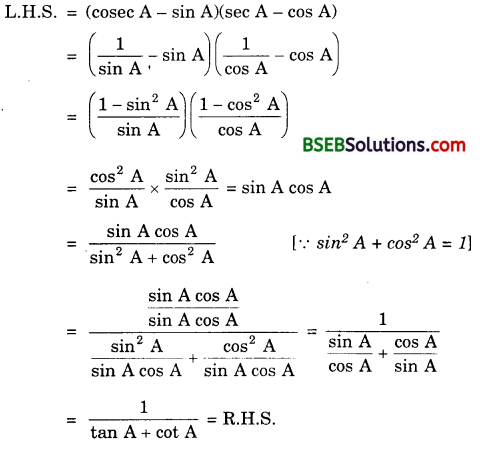(x) We have :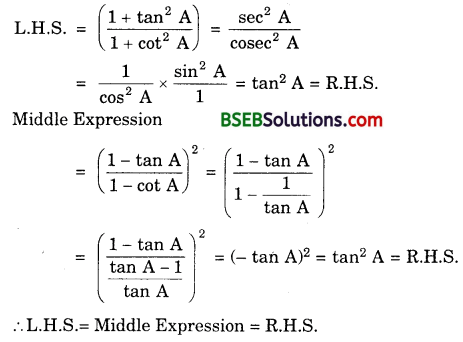## Bihar Board Class 10th Maths Chapter 8 Introduction to Trigonometry Ex 8.4 Textbooks for Exam Preparations

Bihar Board Class 10th Maths Chapter 8 Introduction to Trigonometry Ex 8.4 Textbook Solutions can be of great help in your Bihar Board Class 10th Maths Chapter 8 Introduction to Trigonometry Ex 8.4 exam preparation. The BSEB STD 10th Maths Chapter 8 Introduction to Trigonometry Ex 8.4 Textbooks study material, used with the English medium textbooks, can help you complete the entire Class 10th Maths Chapter 8 Introduction to Trigonometry Ex 8.4 Books State Board syllabus with maximum efficiency.

## FAQs Regarding Bihar Board Class 10th Maths Chapter 8 Introduction to Trigonometry Ex 8.4 Textbook Solutions

#### How to get BSEB Class 10th Maths Chapter 8 Introduction to Trigonometry Ex 8.4 Textbook Answers??

Students can download the Bihar Board Class 10 Maths Chapter 8 Introduction to Trigonometry Ex 8.4 Answers PDF from the links provided above.

#### Can we get a Bihar Board Book PDF for all Classes?

Yes you can get Bihar Board Text Book PDF for all classes using the links provided in the above article.

## Important Terms

Bihar Board Class 10th Maths Chapter 8 Introduction to Trigonometry Ex 8.4, BSEB Class 10th Maths Chapter 8 Introduction to Trigonometry Ex 8.4 Textbooks, Bihar Board Class 10th Maths Chapter 8 Introduction to Trigonometry Ex 8.4, Bihar Board Class 10th Maths Chapter 8 Introduction to Trigonometry Ex 8.4 Textbook solutions, BSEB Class 10th Maths Chapter 8 Introduction to Trigonometry Ex 8.4 Textbooks Solutions, Bihar Board STD 10th Maths Chapter 8 Introduction to Trigonometry Ex 8.4, BSEB STD 10th Maths Chapter 8 Introduction to Trigonometry Ex 8.4 Textbooks, Bihar Board STD 10th Maths Chapter 8 Introduction to Trigonometry Ex 8.4, Bihar Board STD 10th Maths Chapter 8 Introduction to Trigonometry Ex 8.4 Textbook solutions, BSEB STD 10th Maths Chapter 8 Introduction to Trigonometry Ex 8.4 Textbooks Solutions,
Share: